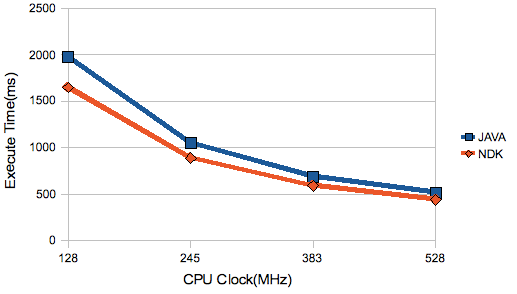How fast is Android NDK? Basic test on NDK!You all may wonder how fast is code written with Native C/C++ (Android NDK). DroidSans moderator, Mr.Kanatorn, has already done some experiment on this by running this simple mathematical calculation code with both Java and Native C on his G1.

for (i=0; i < 100000; i++) {
test=i/(i+1.01f/(i/0.123456));
}

And here is the result.

You will see that Native C/C++ with NDK is faster than Java but not much. This can refer to two things, NDK is not that much efficient or Android Java is quite effective. However this is just the simple test, we need some advance test to prove NDK's efficiency.

All credit go to Mr.Kanatorn. Good job!

You all may wonder how fast is code written with Native C/C++ (Android NDK). DroidSans moderator, Mr.Kanatorn, has already done some experiment on this by running this simple mathematical calculation code with both Java and Native C on his G1.

```for (i=0; i < 100000; i++) {
test=i/(i+1.01f/(i/0.123456));
}
```

And here is the result.

You will see that Native C/C++ with NDK is faster than Java but not much. This can refer to two things, NDK is not that much efficient or Android Java is quite effective. However this is just the simple test, we need some advance test to prove NDK’s efficiency.

All credit go to Mr.Kanatorn. Good job!

To Top